Students can download Maths Chapter 2 Measurements Ex 2.3 Questions and Answers, Notes, Samacheer Kalvi 6th Maths Guide Pdf helps you to revise the complete Tamilnadu State Board New Syllabus, helps students complete homework assignments and to score high marks in board exams.

## Tamilnadu Samacheer Kalvi 6th Maths Solutions Term 2 Chapter 2 Measurements Ex 2.3

Miscellaneous practice problems

Question 1.
Two pipes whose lengths are 7 m 25 cm and 8 m 13 cm joined by welding and then a small piece 60 cm is cut from the whole. What is the remaining length of the pipe?
Solution:
Total length = 7 m 25 cm + 8 m 13 cm = 15 m 38 cm (or) 1538 cm
length detatched = 60 cm
Remaining length = 14 m 78 cmQuestion 2.
The saplings are planted at a distance of 2 m 50 cm in the road of length 5 km by Saravanan. If he has 2560 saplings, how many saplings will be planted by him? how many saplings are left?
Solution:
Distance between two saplings = 2 m 50 cm = 250 cm
Total length of the road = 5000 m = 500000 cm

Question 3.
Put ✓ a mark in the circles which adds upto the given measure.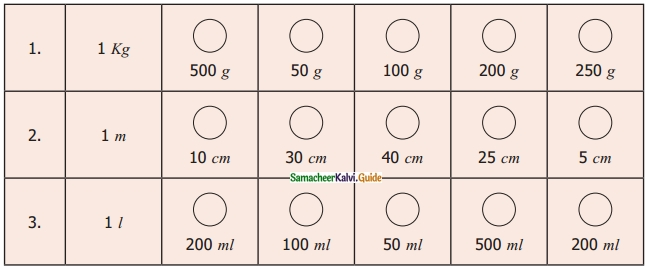Solution: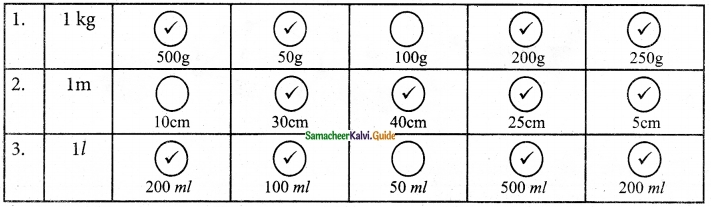Question 4.
Make a calendar for the month of February 2020. (Hint: January 1st, 2020 is Wednesday)
Solution:
February 2020 is a leap year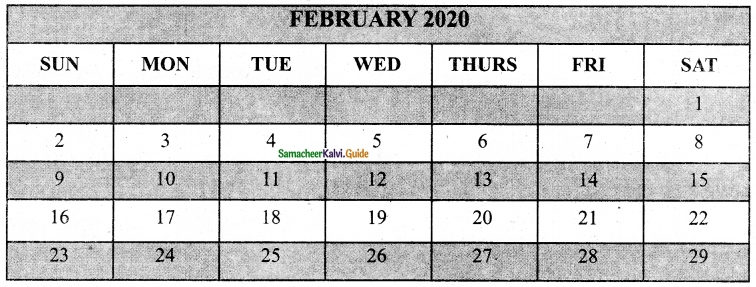Question 5.
Observe and Collect the data for a minute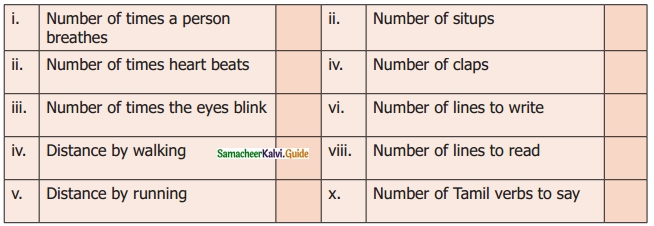Solution:Challenge Problems

Question 6.
A squirrel wants to eat the grains quickly. Help the Squirrel to find the shortest way to reach the grains. (Use your scale to measure the length of the line segments)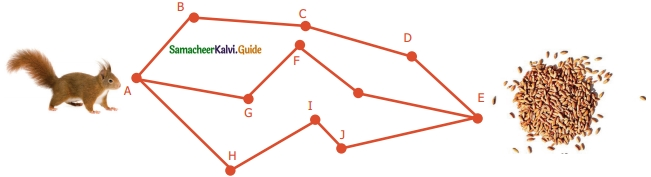Solution: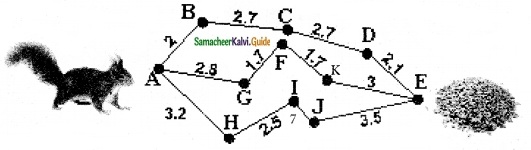The Shortest way to reach the grains is the AGFKE path.

Question 7.
A room has a door whose measures are 1 m wide and 2 m 50 cm high.
Can we make a bed of 2 m and 20 cm in length and 90 cm wide into the room?
Solution:
Measures of the door length 2 m 50 cm width and lm (100 cm)
(i) Measures of the bed 2 m 20 cm width and 90 cm
Measures of the bed < measures of the door
∴ Yes, we can take the bed into the room.Question 8.
A post office functions from 10 a.m. to 5.45 p.m. with a lunch break of 1 hour. If the post office works for 6 days a week, find the total duration of working hours in a week.
Solution:
Working hours in a day = 6 hrs 45 min
= (6 × 60 min) + 45 min
= (360 + 45) min
= 405 min
Total duration of working hours in a week
= 6 × 405 min
= 2430 min
= $$\frac{2430}{60}$$
= $$\frac{810}{20}$$
= 40 $$\frac{1}{2}$$
= 40 hours 30 minutesQuestion 9.
Seetha wakes up at 5.20 a.m. She spends 35 minutes to get ready and travels 15 minutes to reach the railway station. If the train departs exactly at 6.00 am, will Seetha catch the train?
Solution:
No, Seetha will not catch the train
Time at Seetha wakes up = 5.20 am
Time is taken for getting ready = 35 min
Travelling time to station = 15 min
Reporting time = 5.20 am + 50 min = 6.10 am
But, the train departs exactly at 6.00 am
So, Seetha will not catch the train.Question 10.
A doctor advised Vairavan to take one tablet every 6 hours once on the 1st day and once every 8 hours on the 2nd and 3rd day. If he starts to take 9.30 am the first dose. Prepare a time chart to take the tablet in railway time.
Solution: